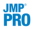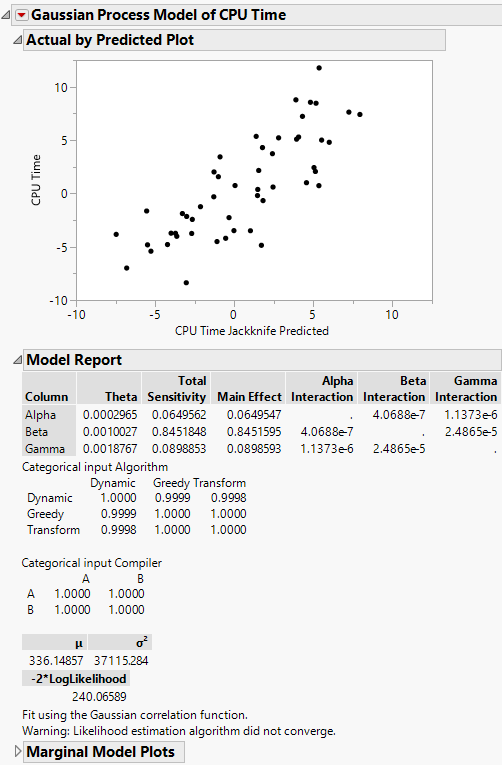Predictive and Specialized Modeling > Gaussian Process > Additional Examples of the Gaussian Process Platform > Example of Gaussian Process Model with Categorical Predictors
Publication date: 04/12/2021

##Example of Gaussian Process Model with Categorical Predictors

This example uses the Algorithm Data.jmp sample data table. These data are simulated CPU times from a 50 run space filling designed experiment. The Algorithm Factors.jmp sample data table provides the factors and settings for the design. The design has three continuous and two categorical factors. The goal is to predict CPU Time using a Gaussian Process model that contains both continuous and categorical factors.

1. Select Help > Sample Data Library and open Design Experiment/Algorithm Data.jmp.

2. Select Analyze > Specialized Modeling > Gaussian Process.

3. Select Alpha through Compiler and click X.

4. Select CPU Time and click Y.

5.To run the analysis, leave the Fast GASP checked. Click OK.

Note: The Fast GASP option must be used for models that contain categorical factors. See Models with Categorical Predictors.

Figure 17.7 Algorithm Data ReportThe actual by predicted plot shows a strong correlation between the actual and predicted CPU times. This in an indication that the Gaussian process prediction model is a good approximation of the true function. In the Model Report, the Beta predictor has the highest total sensitivity. This indicates that of the continuous predictors, Beta explains the most variation in the response. There is a separate Categorical Input matrix for each of the categorical predictors, Algorithm and Compiler. These matrices are correlation matrices and show the correlation between levels for each categorical predictor. The off-diagonals of the matrices are the τ parameters.

Want more information? Have questions? Get answers in the JMP User Community (community.jmp.com).